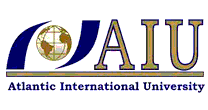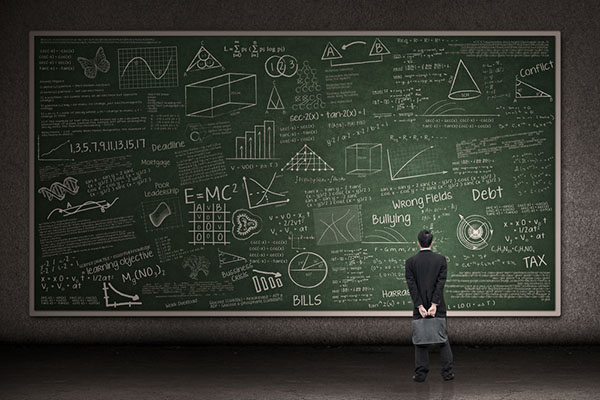﻿ Algebra with Applications - Open Courses by Atlantic International University - AIU#### Algebra with ApplicationsAlgebra with Applications Course

The following course in Algebra with Applications is provided in its entirety by Atlantic International University's "Open Access Initiative " which strives to make knowledge and education readily available to those seeking advancement regardless of their socio-economic situation, location or other previously limiting factors. The University's Open Courses are free and do not require any purchase or registration, they are open to the public.

The course in Algebra with Applications contains the following:

• Lessons in video format with explaination of theoratical content.
• Complementary activities that will make research more about the topic , as well as put into practice what you studied in the lesson. These activities are not part of their final evaluation.
• Texts supporting explained in the video.
• Evaluation questionnaire, that will grant access to the next lesson after approval.
• Final exam for overall evaluation of the course.

The Administrative Staff may be part of a degree program paying up to three college credits. The lessons of the course can be taken on line Through distance learning. The content and access are open to the public according to the "Open Access" and " Open Access " Atlantic International University initiative. Participants who wish to receive credit and / or term certificate , must register as students.

Lesson 1: Mathematics

In Latin, and in English until around 1700, the term mathematics more commonly meant "astrology" (or sometimes "astronomy") rather than "mathematics"; the meaning gradually changed to its present one from about 1500 to 1800. This has resulted in several mistranslations: a particularly notorious one is Saint Augustine's warning that Christians should beware of mathematici meaning astrologers, which is sometimes mistranslated as a condemnation of mathematicians.

Lesson 2: Exponents

Good leaders are made not born. If you have the desire and willpower, you can become an effective leader. Good leaders develop through a never ending process of self-study, education, training, and experience (Jago, 1982). This guide will help you through that process.

To inspire your workers into higher levels of teamwork, there are certain things you must be, know, and, do. These do not come naturally, but are acquired through continual work and study. Good leaders are continually working and studying to improve their leadership skills; they are NOT resting on their laurels.

Lesson 3: Algebra

Algebra (from Arabic al-jebr meaning "reunion of broken parts") is one of the broad parts of mathematics, together with number theory, geometry and analysis. As such, it includes everything from elementary equation solving to the study of abstractions such as groups, rings, and fields. The more basic parts of algebra are called elementary algebra, the more abstract parts are called abstract algebra or modern algebra. Elementary algebra is essential for any study of mathematics, science, or engineering, as well as such applications as medicine and economics. Abstract algebra is a major area in advanced mathematics, studied primarily by professional mathematicians. Much early work in algebra, as the origin of its name suggests, was done in the Near East, by such mathematicians as Omar Khayyam (1050-1123)

Lesson 4: MATRIX

The history of matrices goes back to ancient times! But the term "matrix" was not applied to the concept until 1850. "Matrix" is the Latin word for womb, and it retains that sense in English. It can also mean more generally any place in which something is formed or produced. The origins of mathematical matrices lie with the study of systems of simultaneous linear equations. An important Chinese text from between 300 BC and AD 200, Nine Chapters of the Mathematical Art (Chiu Chang Suan Shu), gives the first known example of the use of matrix methods to solve simultaneous equations.

Lesson 5: Derived

The concept of Derivative is at the core of Calculus and modern mathematics. The definition of the derivative can be approached in two different ways. One is geometrical (as a slope of a curve) and the other one is physical (as a rate of change). Historically there was (and maybe still is) a fight between mathematicians which of the two illustrates the concept of the derivative best and which one is more useful. We will not dwell on this and will introduce both concepts. Our emphasis will be on the use of the derivative as a tool. The Physical Concept of the Derivative This approach was used by Sir Isaac Newton in the development of his Classical Mechanics. The main idea is the concept of velocity and speed. Indeed, assume you are traveling from point A to point B, what is the average velocity during the trip?

Lesson 6: Integral

Integrals and derivatives became the basic tools of calculus, with numerous applications in science and engineering. The founders of the calculus thought of the integral as an infinite sum of rectangles of infinitesimal width. A rigorous mathematical definition of the integral was given by Bernhard Riemann. It is based on a limiting procedure which approximates the area of a curvilinear region by breaking the region into thin vertical slabs. Beginning in the nineteenth century, more sophisticated notions of integrals began to appear, where the type of the function as well as the domain over which the integration is performed has been generalized

Lesson 7: Algebraic Equations

The algebraic equations over the rationals with only one variable are also called univariate equations. They have a very long history. Ancient mathematicians wanted the solutions in the form of radical expressions,. The ancient Egyptians knew how to solve equations of degree 2 in this manner. In the 9th century Muhammad ibn Musa al-Khwarizmi and other Islamic mathematicians derived the general quadratic formula and recognized the importance of the discriminant. During the Renaissance, Gerolamo Cardano found the solution to equations of degree 3 and Lodovico Ferrari solved equations of degree 4. Finally Niels Henrik Abel proved, in 1824, that equations of degree 5 and equations of higher degree are not always solvable using radicals. Galois theory, named after Évariste Galois, was introduced to give criteria deciding if an equation is solvable using radicals.

Lesson 8: Maximus/Minimus

In mathematics, the maximum and minimum (plural: maxima and minima) of a function, known collectively as extrema (singular: extremum), are the largest and smallest value that the function takes at a point either within a given neighborhood (local or relative extremum) or on the function domain in its entirety (global or absolute extremum). Pierre de Fermat was one of the first mathematicians to propose a general technique (called adequality) for finding maxima and minima. More generally, the maximum and minimum of a set (as defined in set theory) are the greatest and least element in the set. Unbounded infinite sets such as the set of real numbers have no minimum and maximum.

Lesson 9: Algebra Theories I

An algebraic theory is a concept in universal algebra that describes a specific type of algebraic gadget, such as groups or rings. An individual group or ring is a model of the appropriate theory. Roughly speaking, an algebraic theory consists of a specification of operations and laws that these operations must satisfy. Traditionally, algebraic theories were described in terms of logical syntax, as first-order theories whose signatures have only function symbols, no relation symbols, and all of whose axioms are universally quantified equations. Such descriptions may be viewed as presentations of a theory, analogous to generators and relations presentations of groups. In particular, different logical presentations can lead to equivalent mathematical objects

Lesson 10: Algebra Theories II

An actual algebraic theory is one where all operations are total functions. The most familiar example may be the (strict) notion of category: a small category consists of a set C0of objects, a set C1 of morphisms, source and target maps s,t:C1→C0 and so on, but composition is only defined for pairs of morphisms where the source of one happens to equal the target of the other. Essentially algebraic theories can be understood through category theory at least when they are finitary, so that all operations have only finitely many arguments. This gives a generalization of Lawvere theories, which describe finitary algebraic theories. As the domains of the operations are given by the solutions to equations, they may be understood using the notion of equalizer. So, just as a Lawvere theory is defined using a category with finite products, a finitary essentially algebraic theory is defined using a category with finite limits — or in other words, finite products and also equalizers (from which all other finite limits, including pullbacks, may be derived).